# A simple Python program for an ANN to cover the MNIST dataset – XIV – cluster detection in feature space

We extend our studies of a program for a Multilayer perceptron and gradient descent in combination with the MNIST dataset:

In this article we shall work a bit on the following topic: How can we reduce the computational time required for gradient descent runs of our MLP?

Readers who followed my last articles will have noticed that I sometimes used 1800 epochs in a gradient descent run. The computational time including

• costly intermediate print outs into Jupyter cells,
• a full determination of the reached accuracy both on the full training and the test dataset at every epoch

lay in a region of 40 to 45 minutes for our MLP with two hidden layers and roughly 58000 weights. Using an Intel I7 standard CPU with OpenBlas
support. And I plan to work with bigger MLPs – not on MNIST but other data sets. Believe me: Everything beyond 10 minutes is a burden. So, I have a natural interest in accelerating things on a very basic level already before turning to GPUs or arrays of them.

# Factors for CPU-time

This introductory question leads to another one: What basic factors beyond technical capabilities of our Linux system and badly written parts of my Python code influence the consumption of computational time? Four points come to my mind; you probably find even more:

• One factor is certainly the extra forward propagation run which we apply to all samples of both the test and training data seat the end of each epoch. We perform this propagation to make predictions and to get data on the evolution of the accuracy, the total loss and the ratio of the regularization term to the real costs. We could do this in the future at every 2nd or 5th epoch to save some time. But this will reduce CPU-time only by less than 22%. 76% of the CPU-time of an epoch is spent in batch-handling with a dominant part in error backward propagation and weight corrections.
• The learning rate has a direct impact on the number of required epochs. We could enlarge the learning rate in combination with input data normalization; see the last article. This could reduce the number of required epochs significantly. Depending on the parameter choices before by up to 40% or 50%. But it requires a bit of experimenting ….
• Two other, more important factors are the frequent number of matrix operations during error back-propagation and the size of the involved matrices. These operations depend directly on the number of nodes involved. We could therefore reduce the number of nodes of our MLP to a minimum compatible with the required accuracy and precision. This leads directly to the next point.
• The dominant weight matrix is of course the one which couples layer L0 and layer L1. In our case its shape is 784 x 70; it has almost 55000 elements. The matrix for the next pair of layers has only 70×30 = 2100 elements – it is much, much smaller. To reduce CPU time for forward propagation we should try to make this matrix smaller. During error back propagation we must perform multiple matrix multiplications; the matrix dimensions depend on the number of samples in a mini-batch AND on the number of nodes in the involved layers. The dimensions of the the result matrix correspond to the those of the weight matrix. So once again: A reduction of the nodes in the first 2 layers would be extremely helpful for the expensive backward propagation. See: The math behind EBP.

# Reduction of the dimensions of the dominant matrix“requires a reduction of input features

The following numbers show typical CPU times spend for matrix operations during error back propagation [EBP] between different layers of our MLP and for two different batches at the beginning of gradient descent:

```Time_CPU for BW layer operations (to L2) 0.00029015699965384556
Time_CPU for BW layer operations (to L1) 0.0008645610000712622
Time_CPU for BW layer operations (to L0) 0.006551215999934357

Time_CPU for BW layer operations (to L2) 0.00029157400012991275
Time_CPU for BW layer operations (to L1) 0.0009575330000188842
Time_CPU for BW layer operations (to L0) 0.007488838999961445
```

The operations involving layer L0 cost a factor of 7 more CPU time than the other operations! Therefore, a key to the reduction of the number of mathematical operations is obviously the reduction of the number of nodes in the input layer! We cannot reduce the numbers in the hidden layers much, if we
do not want to hamper the accuracy properties of our MLP too much. So the basic question is

Can we reduce the number of input nodes somehow?

Yes, maybe we can! Input nodes correspond to „features„. In case of the MNIST dataset the relevant features are given by the gray-values for the 784 pixels of each image. A first idea is that there are many pixels within each MNIST image which are probably not used at all for classification – especially pixels at the outer image borders. So, it would be helpful to chop them off or to ignore them by some appropriate method. In addition, special significant pixel areas may exist to which the MLP, i.e. its weight optimization, reacts during training. For example: The digits 3, 5, 6, 8, 9 all have a bow within the lower 30% of an image, but in other regions, e.g. to the left and the right, they are rather different.

If we could identify suitable image areas in which dark pixels have a higher probability for certain digits then, maybe, we could use this information to discriminate the represented digits? But a „higher density of dark pixels in an image area“ is nothing else than a description of a „cluster“ of (dark) pixels in certain image areas. Can we use pixel clusters at numerous areas of an image to learn about the represented digits? Is the combination of (averaged) feature values in certain clusters of pixels representative for a handwritten digit in the MNIST dataset?

If the number of such pixel clusters could be reduced below lets say 100 then we could indeed reduce the number of input features significantly!

# Cluster detection

To be able to use relevant „clusters“ of pixels – if they exist in a usable form in MNIST images at all – we must first identify them. Cluster identification and discrimination is a major discipline of Machine Learning. This discipline works in general with unlabeled data. In the MNIST case we would not use the labels in the „y“-data at all to identify clusters; we would only use the „X“-data. A nice introduction to the mechanisms of cluster identification is given in the book of Paul Wilcott (see Machine Learning – book recommendations for the reference). The most fundamental method – called „kmeans“ – iterates over 3 major steps [I simplify a bit :-)]:

• We assume that K clusters exist and start with random initial positions of their centers (called „centroids“) in the multidimensional feature space
• We measure the distance of all data points to he centroids and associate a point with that centroid to which the distance is smallest
• We determine the „center of mass“ (according to some distance metric) of the identified data point groups and assume it as a new position of the centroids and move the old positions (a bit) in this direction.

We iterate over these steps until the centroids‘ positions hopefully get stable. Pretty simple. But there is a major drawback: You must make an assumption on the number „K“ of clusters. To make such an assumption can become difficult in the complex case of a feature space with hundreds of dimensions.

You can compensate this by executing multiple cluster runs and comparing the results. By what? Regarding the closure or separation of clusters in terms of an appropriate norm. One such norm is called „cluster inertia„; it measures the mean squared distance to the center for all points of a cluster. The theory is that the sum of the inertias for all clusters drops significantly with the number of clusters until an optimal number is reached and the inertia curve flattens out. The point where this happens in a plot of inertia vs. number of clusters is called „elbow„.
Identifying this „elbow“ is one of the means to find an optimal number of clusters. However, this recipe does not work under all circumstances. As the number of clusters get big we may be confronted with a smooth decline of the inertia sum.

# What data do we use for gradient descent after cluster detection?

How could we measure whether an image shows certain clusters? We could e.g. measure distances (with some appropriate metric) of all image points to the clusters. The „fit_transform()“-method of KMeans and MiniBatchKMeans provide us with with some distance measure of each image to the identified clusters. This means our images are transformed into a new feature space – namely into a „cluster-distance space“. This is a quite complex space, too. But it has less dimensions than the original feature space!

Note: We would of course normalize the resulting distance data in the new feature space before applying gradient descent.

# Application of „KMeansBatch“ to MNIST

There are multiple variants of „KMeans“. We shall use one which is provided by SciKit-Learn and which is optimized for large datasets: „MiniBatchKMeans„. It operates batch-wise without loosing too much of accuracy and convergence properties in comparison to KMeans (or a comparison see here). „MiniBatchKMeans“has some parameters you can play with.

We could be tempted to use 10 clusters as there are 10 digits to discriminate between. But remember: A digit can be written in very many ways. So, it is much more probable that we need a significant larger number of clusters. But again: How to determine on which K-values we should invest a bit more time? „Kmeans“ and methods alike offer another quantity called „silhouette“ coefficient. It measures how well the data points are within, at or outside the borders of a cluster. See the book of Geron referenced at the link given above on more information.

# Variation of CPU time, inertia and average silhouette coefficients with the number of clusters „K“

Let us first have a look at the evolution of CPU time, total inertia and averaged silhouette with the number of clusters „K“ for two different runs. The following code for a Jupyter cell gives us the data:

```
# *********************************************************
# Pre-Clustering => Searching for the elbow
# *********************************************************
from sklearn.cluster import KMeans
from sklearn.cluster import MiniBatchKMeans
from sklearn.preprocessing import StandardScaler
from sklearn.metrics import silhouette_score
X = np.concatenate((ANN._X_train, ANN._X_test), axis=0)
y = np.concatenate((ANN._y_train, ANN._y_test), axis=0)
print("X-shape = ", X.shape, "y-shape = ", y.shape)
num = X.shape

li_n = []
li_inertia = []
li_CPU = []
li_sil1 = []

# Loop over the number "n" of assumed clusters
rg_n = range(10,171,10)
for n in rg_n:
print("\nNumber of clusters: ", n)
start = time.perf_counter()
kmeans = MiniBatchKMeans(n_clusters=n, n_init=500, max_iter=1000, batch_size=500 )
X_clustered = kmeans.fit_transform(X)
sil1 = silhouette_score(X, kmeans.labels_)
#sil2 = silhouette_score(X_clustered, kmeans.labels_)
end = time.perf_counter()
dtime = end - start
print('Inertia = ', kmeans.inertia_)
print('Time_CPU = ', dtime)
print('sil1 score = ', sil1)
li_n.append(n)
li_inertia.append(kmeans.inertia_)
li_CPU.append(dtime)
li_sil1.append(sil1)

# Plots
# ******
fig_size = plt.rcParams["figure.figsize"]
fig_size[
0] = 14
fig_size = 5
fig1 = plt.figure(1)
fig2 = plt.figure(2)

ax1_1.plot(li_n, li_CPU)
ax1_1.set_xlabel("num clusters K")
ax1_1.set_ylabel("CPU time")

ax1_2.plot(li_n, li_inertia)
ax1_2.set_xlabel("num clusters K")
ax1_2.set_ylabel("inertia")

ax2_1.plot(li_n, li_sil1)
ax2_1.set_xlabel("num clusters K")
ax2_1.set_ylabel("silhoutte 1")

```

You see that I allowed for large numbers of initial centroid positions and iterations to be on the safe side. Before you try it yourself: Such runs for a broad variation of K-values are relatively costly. The CPU time rises from around 32 seconds for 30 clusters to a little less than 1 minute for 180 clusters. These times add up to a significant sum after a while …

Here are some plots: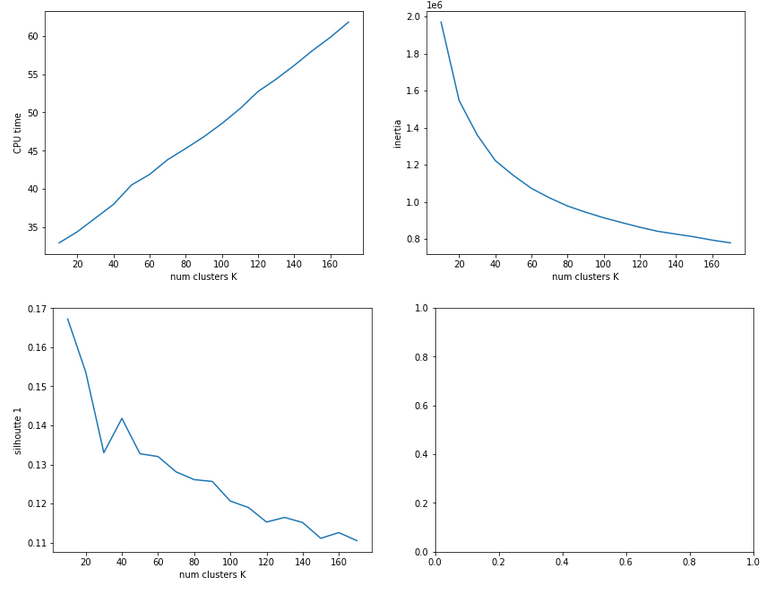The second run was executed with a higher resolution of K_(n+1) – K_n 5 = 5.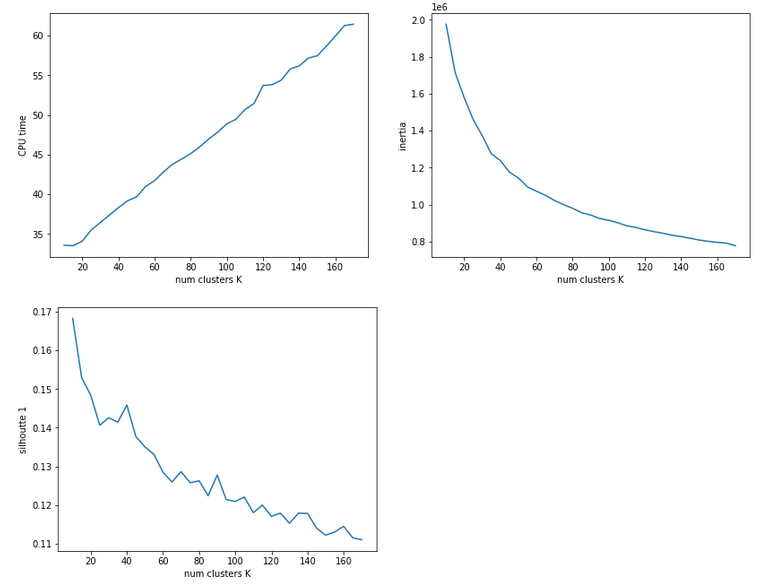We see that the CPU time to determine the centroids‘ positions varies fairly linear with „K“. And even for 170 clusters it does not take more than a minute! So, CPU-time for cluster identification is not a major limitation.

Unfortunately, we do not see a clear elbow in the inertia curve! What you regard as a reasonable choice for the number K depends a lot on where you say the curve starts to flatten. You could say that this happens around K = 60 to 90. But the results for the silhouette-quantity indicate for our parameter setting that K=40, K=70, K=90 are interesting points. We shall look at these points a bit closer with higher resolution later on.

# Reduction of the regularization factor (for Ridge regularization)

Now, I want to discuss an important point which I did not find in the literature:
In my last article we saw that regularization plays a significant but also delicate role in reaching top accuracy values for the test dataset. We saw that Lambda2 = 0.2 was a good choice for a normalized input of the MNIST data. It corresponded to a certain ratio of the regularization term to average batch costs.
But when we reduce the number of input nodes we also reduce the number of total weights. So the weight values themselves will automatically become bigger if we want to get to similar good values at the second layer. But as the regularization term depends in a quadratic way on the weights we may assume that we roughly need a linear reduction of Lambda2. So, for K=100 clusters we may shrink Lambda2 to (0.2/784*100) = 0.025 instead of 0.2. In general:

Lambda2_cluster = Lambda2_std * K / (number of input nodes)

I applied this rule of a thumb successfully throughout experiments with clustering befor gradient descent.

# Reference run without clustering

We saw at the end of article XII that we could reach an accuracy of around 0.975 after 500 epochs under optimal circumstances. But in the case I presented ten I was extremely lucky with the statistical initial weight distribution and the batch composition. In other runs with the same parameter setup I got smaller accuracy values. So, let us take an ad hoc run with the following parameters and results:
Parameters: learn_rate = 0.001, decrease_rate = 0.00001, mom_rate = 0.00005, n_size_mini_batch = 500, n_epochs = 600, Lambda2 = 0.2, weights at
all layers in [-2*1.0/sqrt(num_nodes_layer), 2*1.0/sqrt(num_nodes_layer)]
Results: acc_train: 0.9949 , acc_test: 0.9735, convergence after ca. 550-600 epochs

The next plot shows (from left to right and the down) the evolution of the costs per batch, the averaged error of the last mini-batch during an epoch, the ratio of regularization to batch costs and the total costs of the training set, respectively .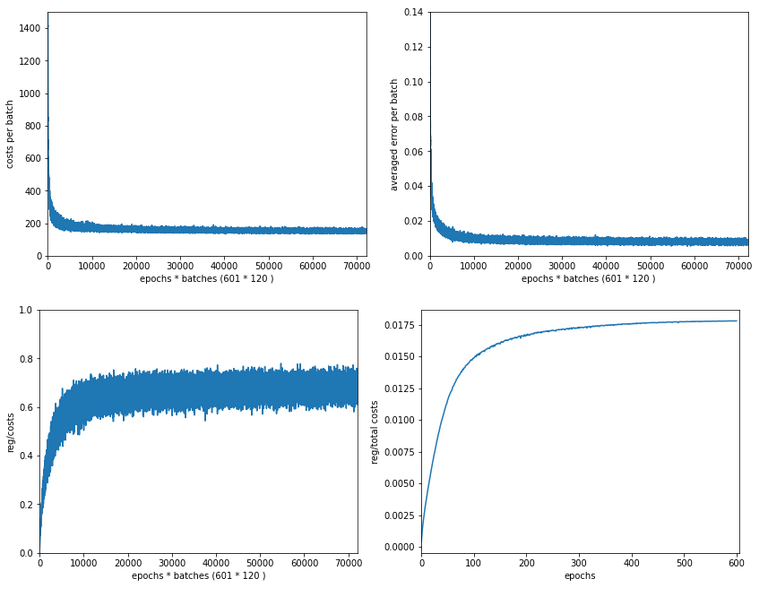The following plot summarizes the evolution of the total costs of the traaining set (including the regularization contribution) and the evolution of the accuracy on the training and the test data sets (in orange and blue, respectively).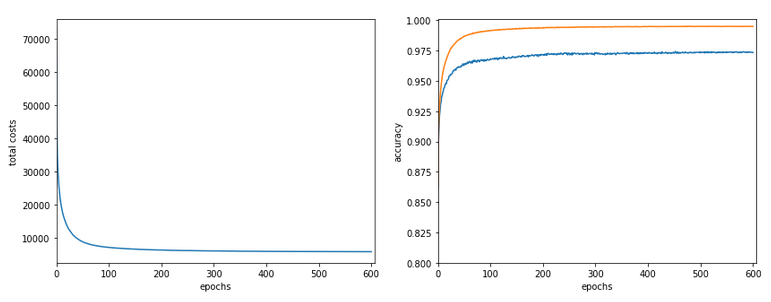The required computational time for the 600 epochs was roughly 18,2 minutes.

# Results of gradient descent based on a prior cluster identification

Before we go into a more detailed discussion of code adaption and test runs with things like clusters in unnormalized and normalized feature spaces, I want to show what we – without too much effort – can get out of using cluster detection ahead of gradient descent. The next plot shows the evolution of a run for K=70 clusters in combination with a special normalization: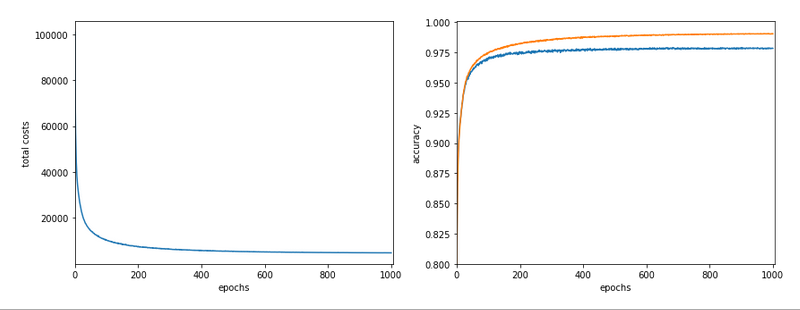and the total cost and accuracy evolution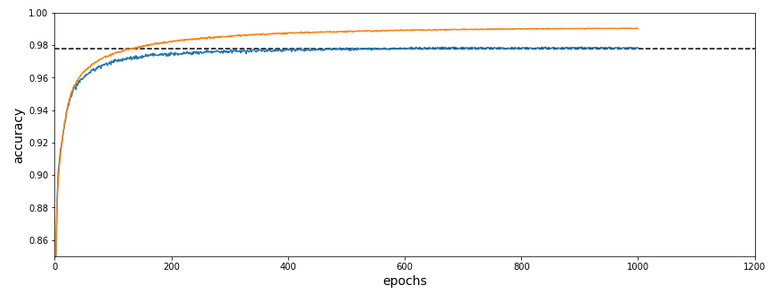The dotted line marks an accuracy of 97.8%! This is 0.5% bigger then our reference value of 97.3%. The total gain of %gt; 0.5% means however 18.5% of the remaining difference of 2.7% to 100% and we past a value of 97.8% already at epoch 600 of the run.

What were the required computational times?

If we just wanted 97.4% as accuracy we need around 150 epochs. And a total CPU time of 1.3 minutes to get to the same accuracy as our reference run. This is a factor of roughly 14 in required CPU time. For a stable 97.73% after epoch 350 we were still a factor of 5.6 better. For a stable accuracy beyond 97.8% we needed around 600 epochs – and still were by a factor of 3.3 faster than our reference run! So, clustering really brings some big advantages with it.

# Conclusion

In
this article I discussed the idea of introducing cluster identification in the (unnormalized or normalized) feature space ahead of gradient descent as a possible means to save computational time. A preliminary trial run showed that we indeed can become significantly faster by at least a factor of 3 up to 5 and even more. This is just due to the point that we reduced the number of input nodes and thus the number of mathematical calculations during matrix operations.

In the next article we shall have a more detailed look at clustering techniques in combination with normalization.